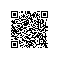# JQuery入门(6)

<script type="text/javascript">
$(function(){$("p").one("click",function(){
$(this).animate({fontSize:"+=6px"}); }); }) </script> <h3>one()方法的使用</h3> <p>这是一个段落。</p> <p>这是另一个段落。</p> <p>请点击 p 元素增加其内容的文本大小。每个 p 元素只会触发一次改事件。</p> 二、手动触发指定事件 trigger() 方法触发被选元素的指定事件类型。 语法形式：$(selector).trigger(event,[param1,param2,…])

<script type="text/javascript">
$(function(){$("div").bind("append-content",function(){
$(this).append("<b>，那是我逝去的青春</b>"); });$("div").trigger("append-content");
})
</script>
...省略代码
<h3>替换元素</h3>
<h3>trigger()手动触发事件</h3>
<div>在夕阳下奔跑</div>

<script type="text/javascript">
$(function(){$("input").focus(function(){//获得焦点
$("input").css("background-color","#FFFFCC"); });$("input").blur(function(){//失去焦点
$("input").css("background-color","#D6D6FF"); }); }) </script> ...省略代码 <h3>焦点事件</h3> 输入名字: <input type="text"> <p>请在上面的输入域中点击，使其获得焦点，然后在输入域外面点击，使其失去焦点。</p> 四、change()改变事件 当元素的值发生改变时，会发生 change 事件。该事件仅适用于文本域（text field），以及 textarea 和 select 元素。change() 函数触发 change 事件，或规定当发生 change 事件时运行的函数。 语法形式：$(selector).change(function)

<script type="text/javascript">
$(function(){$(".field").change(function(){
\$(this).css("background-color","#FFFFCC");
});
})
</script>
...省略代码
<p>在某个域被使用或改变时，它会改变颜色。</p>

<p>性别:
<select class="field" name="male">
<option value="volvo">男</option>
<option value="saab">女</option>
<option value="fiat">中</option>
<option value="audi">保密</option>
</select>
</p>使用钉钉扫一扫加入圈子
+ 订阅